# Volume of a Cube

In this worksheet students will be able to calculate the volume of a cube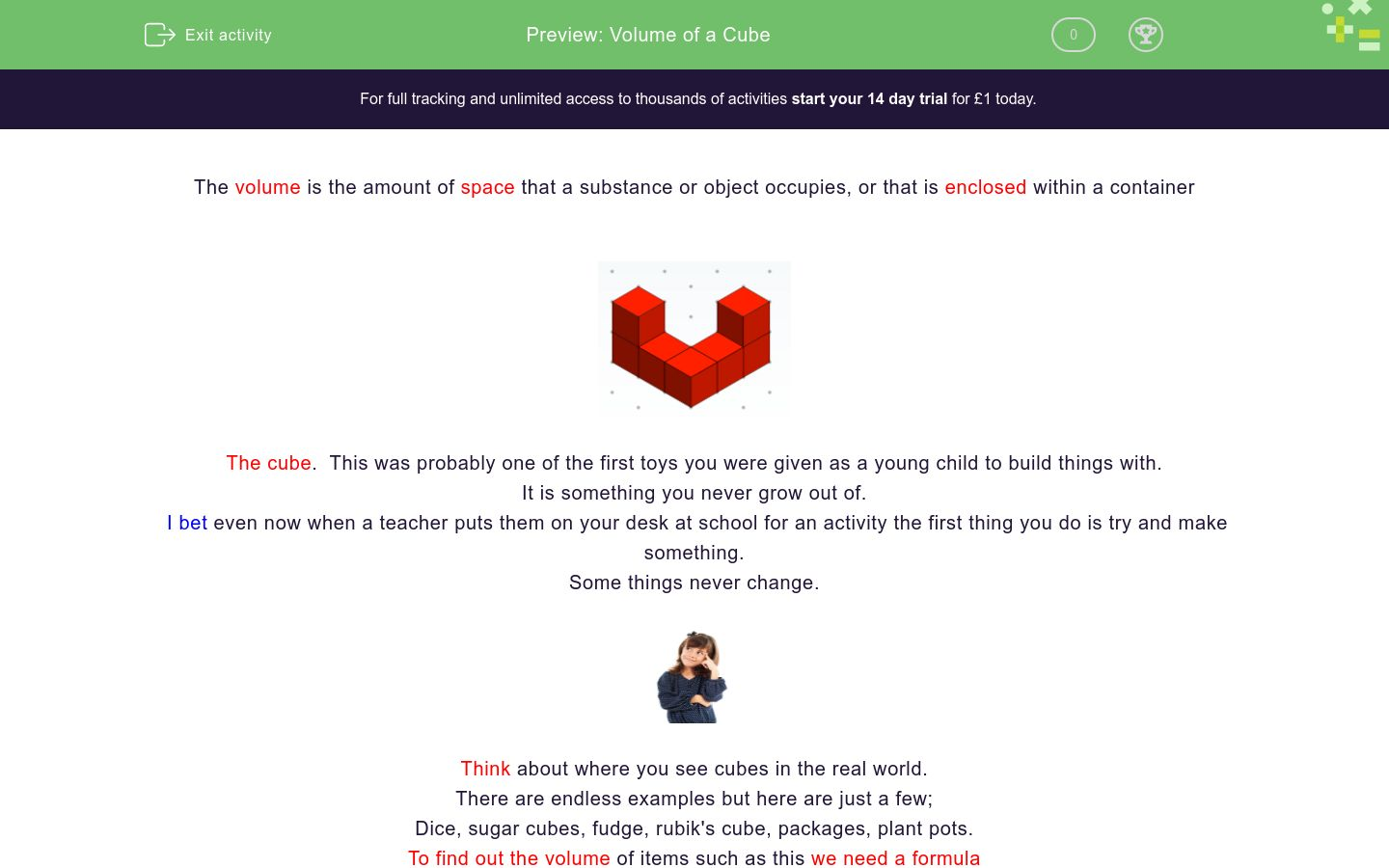Key stage:  KS 4

GCSE Subjects:   Maths

GCSE Boards:   AQA, Eduqas, Pearson Edexcel, OCR

Curriculum topic:   Geometry and Measures, Mensuration

Curriculum subtopic:   Mensuration and Calculation, Volume and Surface Area Calculations

Difficulty level:### QUESTION 1 of 10

The volume is the amount of space that a substance or object occupies, or that is enclosed within a containerThe cube.  This was probably one of the first toys you were given as a young child to build things with.

It is something you never grow out of.

I bet even now when a teacher puts them on your desk at school for an activity the first thing you do is try and make something.

Some things never change.Think about where you see cubes in the real world.

There are endless examples but here are just a few;

Dice, sugar cubes, fudge, rubik's cube, packages, plant pots.

To find out the volume of items such as this we need a formulaBecause a cube is 3 dimensional we are looking for three measurements as shown above.

Volume of a cube is length x length x length

sometimes written as base x length x heightSquares have sides that are all the same length.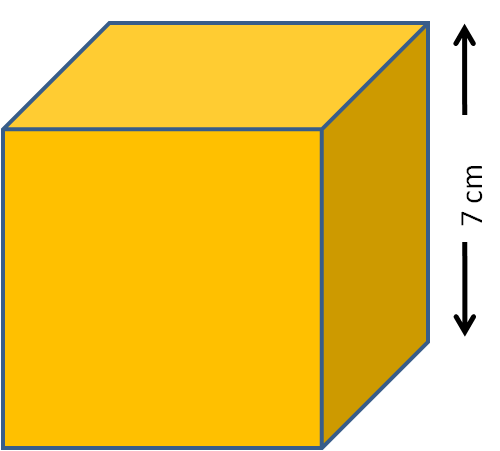Volume of this cube = 7 x 7 x 7 = 343 cm³

We measure volume in units cubed.

On your calculator, you can do 7 then press x³ button.

Try the activities by putting all three numbers in so that you are 100% sure you are confident in what you are doing.

You may not always be allowed a calculator for this type of question.Warning - An easy mistake is 7 x 3 =21 cm³.  As you can see this is a totally different answer.Find the volume of this cube.

Match up the volume of the following.

Answers have been rounded to 2 decimal places when appropriate.

## Column B

Cube with side 4 cm
3.38 cm³
Cube with side 6 cm
512 cm³
Cube with side 3.5 cm
64 cm³
Cube with side 2.5 cm
216 cm³
Cube with side 1.5 cm
42.88 cm³
Cube side 8 cm
15.65 cm³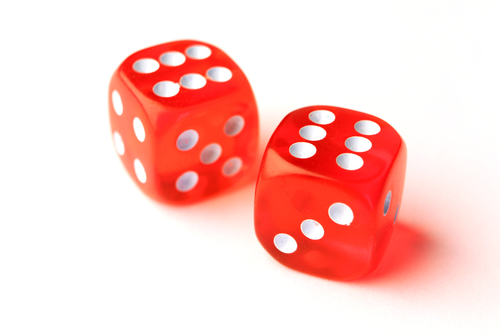What is the total volume of these dice?

The sides are 9 mm.

729 mm²

729 mm³

27 mm³

27 mm²The side of this cube is 3.5 cm.

729 mm²

729 mm³

27 mm³

27 mm²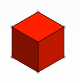The red cube has side 5 cm and the yellow cube has side 8 cm.

What is the difference in their volume?

729 mm²

729 mm³

27 mm³

27 mm²The red cube has side 6 cm and the yellow cube has side 3.5 cm.

 216 cm³ 18 cm³ 42.90 cm³ 42.88 cm³ 173.12cm³ 173.21cm³ Volume of red cube Volume of yellow cube Difference in Volume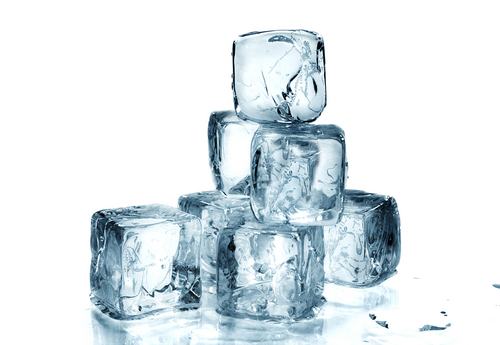In a glass are 7 ice cubes melting.  4 ice cubes have side 2 cm and 3 ice cubes have side 3 cm.

What is the total volume of water once the ice cubes have all melted?

 216 cm³ 18 cm³ 42.90 cm³ 42.88 cm³ 173.12cm³ 173.21cm³ Volume of red cube Volume of yellow cube Difference in VolumeA wooden crate with side 1m is used to transport small parcels, in the shape of a cube.

Each parcel has side 0.5m.

How many small packages will fit inside the crate.

4

6

8

10This cube has side of 2x.  What is its volume?

6x³

8x³

(2x)x (2x) x (2x)cm³

(2x+ (2x)+ (2x)cm³This cube has a volume of 512 cm³

What is the length of one side?

• Question 1Find the volume of this cube.

125
EDDIE SAYS
Just apply the formula. Length x length x length 5 x 5 x 5 = 125 cm³ You wouldn't assemble flat pack furniture without reading the instructions first would you? Okay maybe you would. Following formulas (instructions) in maths is extremely helpful.
• Question 2

Match up the volume of the following.

Answers have been rounded to 2 decimal places when appropriate.

## Column B

Cube with side 4 cm
64 cm³
Cube with side 6 cm
216 cm³
Cube with side 3.5 cm
42.88 cm³
Cube with side 2.5 cm
15.65 cm³
Cube with side 1.5 cm
3.38 cm³
Cube side 8 cm
512 cm³
EDDIE SAYS
Applying the instructions does help, doesn't it? Easy stuff, remember it's the same number multiplied together 3 times. 4 x 4 x 4 6 x 6 x 6 3.5 x 3.5 x 3.5 2.5 x2.5 x 2.5 1.5 x 1.5 x 1.5 8 x 8 x 8
• Question 3What is the total volume of these dice?

The sides are 9 mm.

729 mm³
EDDIE SAYS
Did you remember 9 x 9 x 9 and NOT 9 x 3? Also did you remember the answer for volume is always units³ You are rolling on nicely.
• Question 4The side of this cube is 3.5 cm.

EDDIE SAYS
It is important to get into the habit of always showing your working out. Don't leave it until the exam period to start getting in good habits! If you are not used to doing it in your daily work, you are unlikely to remember to write it in an exam. 3.5 x 3.5 x 3.5 = 42.875 (42.88 to 2 d.p)
• Question 5The red cube has side 5 cm and the yellow cube has side 8 cm.

What is the difference in their volume?

EDDIE SAYS
This is just a way to make sure you are secure at calculating volume and sneaking in an added extra. The word difference should alert you to the fact that a subtraction is required. 5 x 5 x 5 = 125 8 x 8 x 8 = 512 512 - 125 = 387cm ³
• Question 6The red cube has side 6 cm and the yellow cube has side 3.5 cm.

 216 cm³ 18 cm³ 42.90 cm³ 42.88 cm³ 173.12cm³ 173.21cm³ Volume of red cube Volume of yellow cube Difference in Volume
EDDIE SAYS
Length x length x length I hear you shout. Good job. Once you have learnt this this rest is perfection. 6 x 6 x 6 = 216 3.5 x 3.5 x 3.5 = 42.88 216 - 42.88 = 173.12 cm³ I hope you didn't transpose the decimal places and ended up with 173.21 cm ³. Easily done. take extra care when working with decimals.
• Question 7In a glass are 7 ice cubes melting.  4 ice cubes have side 2 cm and 3 ice cubes have side 3 cm.

What is the total volume of water once the ice cubes have all melted?

EDDIE SAYS
Those little added extras, don't you just love them Just think things through carefully and apply what you know. 2 x 2 x 2 = 8 8 x 4 = 32 3 x 3 x 3 = 27 27 x 3 = 81 81 + 32 = 113 cm³
• Question 8A wooden crate with side 1m is used to transport small parcels, in the shape of a cube.

Each parcel has side 0.5m.

How many small packages will fit inside the crate.

8
EDDIE SAYS
Oh of course...the curved ball. First find the volume of the crate. 1 x 1 x 1 = 1 m³ Next find the volume of 1 package 0.5 x0.5 x 0.5 = 0.125 m³ The key word here is INSIDE. This should lead you to 'how many go into' (division) 1 ÷ 0.125 = 8
• Question 9This cube has side of 2x.  What is its volume?

8x³
(2x)x (2x) x (2x)cm³
EDDIE SAYS
Length x length x length, but there are no numbers eeeek. It doesn't matter. Write what you know (2x) x (2x) x (2x) or 8xcm ³.
• Question 10This cube has a volume of 512 cm³

What is the length of one side?

8
8 cm
8cm
EDDIE SAYS
Those curved balls just keep coming. You love them really. You have all the information you need to crack this. Volume = Length x Length x Length (or length³) Do the opposite. The opposite of cm³ is ³√512 ³√512 = 8 Test it out 8 x 8 x 8 = 512 cm³
---- OR ----

Sign up for a £1 trial so you can track and measure your child's progress on this activity.

### What is EdPlace?

We're your National Curriculum aligned online education content provider helping each child succeed in English, maths and science from year 1 to GCSE. With an EdPlace account you’ll be able to track and measure progress, helping each child achieve their best. We build confidence and attainment by personalising each child’s learning at a level that suits them.

Get started